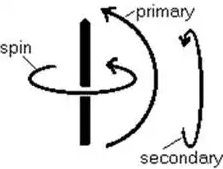Courses

# Study Notes for Gyroscope Notes | EduRev

## Mechanical Engineering : Study Notes for Gyroscope Notes | EduRev

The document Study Notes for Gyroscope Notes | EduRev is a part of the Mechanical Engineering Course Theory of Machines (TOM).
All you need of Mechanical Engineering at this link: Mechanical Engineering

Gyroscope

A gyroscope has three axes. First, a spin axis, which defines the gyroscope strength or moment. Let us call the other two the primary axis and the secondary axis. These three axis are orthogonal to each other.• The spin axis rotates around the vertical line. The primary axis rotates the whole gyroscope in the plane of the page, and the secondary axis rotates the gyroscope up-and-over into the page.
• The spin axis is the source of the gyroscopic effect.
• The primary axis is conceptually the input or driving axis, and the secondary the output. Then if the gyroscope is spun on its spin axis, and a torque is applied to the primary axis, the secondary axis will precess.
• The primary axis appears infinitely stiff to the applied torque and does not give under it. This is the generally recognized characteristic of gyroscopic behavior.
• When a mass ‘m’ moves in a straight line at velocity ‘v’ it exhibits linear momentum (m.v). It is trivial to predict that if it is constrained to travel in a radius ‘r’ it will produce an angular momentum (m.v.r).
• However with the angular momentum, an effect that could not have been predicted turns up - gyroscopic behavior.
• The fact that in the larger world the two effects occur together and in simple proportion to each other does not mean that this is always the case - gyroscopic behaviour occurs without angular momentum in electron behaviour, even though the terms ‘spin’ and ‘spin angular momentum’ are still used for historical reasons, even though there is no direct evidence that the electron’s mass or charge spins on its own axis. It may simply be that rotating an object exposes the gyroscopic moments of the elementary particles that make it up, possibly through the asymmetric relativistic effects created by the centripetal acceleration; some major experimental work is required in this area.
• Angular momentum has the form “kilogram-meters2 per second”.
• Gyroscopic moment has the form “Newton-meters per Hertz”, or torque required to produce a precession rate of one Hertz.
• Both have the dimensions ‘L2M/T’, which means only that they are related by a simple scalar number.
Basic Gyroscope Equations

The strength of a gyroscopic effect is termed the gyroscopic moment. I use the symbol ‘G’, in units “Newton-meters/Hertz”.
A higher moment requires more torque to precess at the same frequency, or for the same torque precesses at a lower rate where a gyroscope receives torque on the primary axis and precession on the secondary, no work is being done.
The torque ‘TP’ on the primary axis has no precession associated with it, while the precession rate ‘vS’ on the secondary axis is:
vS = TP / G
and has no torque associated with it. Since the rate of doing work on each axis is the torque times the precession on that axis, it follows that in this simple case no energy is involved.
Gyroscopes do not differentiate between primary and secondary axes - this is a purely artificial definition of my own.
A torque on the secondary axis creates precession on the primary axis.
Simultaneous torque on both axis will result in simultaneous precession. In this case each axes will have both torque (creating precession on the other axis) and precession (created by torque on the other axis).
Then the rate of doing work ‘PP’ on the primary axis is:
PP = TP.vP / G
and on the secondary:
PS = TS.vS / G
Now by applying the conservation of energy:
PP = - PS
i.e. the work done on one axis must appear on the other.
First apply a forcing torque to the primary axis; at this stage in the argument imagine that the primary axis presents no stiffness against the forcing torque.
The secondary axis would precess at an infinite frequency, but for a limiting mechanism that comes into play; just as torque creates precession, so precession creates torque.
So as the secondary axis precesses it creates a reverse torque TPF on the primary axis:
TPF = -vS.G
The precession rate always runs at that point where TPF is exactly equal and opposite to TP. At this point:
TPF = -vS.G
= - (TP / G).G
= - TP
The reverse torque generated by the precession exactly opposes the applied torque so that the net torque is zero. If it was more the work would be done by the gyroscope. If it was less the primary axis would give way under the applied torque and work would be done with no outlet for it. Both conditions violate conservation of energy principles.
If the secondary axis is locked against rotation and the primary axis is driven, no opposing torque will appear on the primary axis, it is free to rotate without hindrance.
No work is transferred through the gyroscope; there is motion without torque on the primary axis.
The secondary axis has no motion; it is locked but instead experiences a torque TSF:
TSF = vP.G

Offer running on EduRev: Apply code STAYHOME200 to get INR 200 off on our premium plan EduRev Infinity!

## Theory of Machines (TOM)

94 videos|41 docs|28 tests

,

,

,

,

,

,

,

,

,

,

,

,

,

,

,

,

,

,

,

,

,

;